# Classroom

One-eighth of 9th class was interested in studying at a grammar school, at a business academy one sixth, at secondary vocational schools quarter, to SOU one third and the remaining three students were interested in the school of art direction. How many students are in the classroom?

n =  24

### Step-by-step explanation:

n/8+n/6+n/4+n/3+3 = n

3n = 72

n = 24

Our simple equation calculator calculates it.Did you find an error or inaccuracy? Feel free to write us. Thank you!Tips to related online calculators
Need help to calculate sum, simplify or multiply fractions? Try our fraction calculator.
Do you have a linear equation or system of equations and looking for its solution? Or do you have a quadratic equation?

## Related math problems and questions:

• Secondary school1/2 of the pupils want to study at the secondary school, 1/4 at the apprentice, 1/6 at the grammar school 3 pupils do not want to study. How many students are in the class?
• Class 8.AThree quarters of class 8.A went skiing. Of those who remained at home one third was ill and the remaining six were on math olympic. How many students have class 8.A?
• Dentist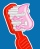Class 5. A participated in a preventive dental examination. A quarter of the pupils found two dental caries, an eighth of the pupils found one caries. Half the class had all their teeth healthy. How many students attend class 5. A if 4 students were missi
• Class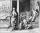When Pythagoras asked how many students attend the school, he said: "Half of the students studying mathematics, 1/4 music, seventh silent, and there are three girls at school." How many students had Pythagoras at school?
• The classroom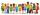In the seventh class the number of boys and girls are at a ratio of 4:5. Boys are 16. How many total students are in the classroom?
• ShirtsIn a classroom, 1/6 of the students are wearing blue shirts and 2/3 are wearing white shirts. There are 36 students in the class. How many students are wearing a shirt other than blue or white?
• SchoolsThree schools are attended by 678 pupils. To the first attend 21 students more and to the third 108 fewer students than to second school. How many students attend the schools?
• Shirts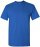In a classroom,1/6 of the students are wearing blue shirts, and 2/3 are wearing white shirts. There are 18 students in the classroom. How many students are wearing shirts other than blue shirts or white shirts?
• English and math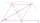1/5 of a class study English, and 3/5 study mathematics. If the class consist of 80 students, how many students study English and mathematics in the class?
• Summerjobs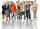The agency give summerjobs for 2352 students in 2018. The eighth was high-school students, the rest was undergraduates. How many undergraduates works via agency in 2018?
• Classroom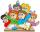In a class are 32 pupils. Boys are 8 less than girls. How many boys and girls are in the classroom?
• BirthdaysIn the classroom, students always give candy to their classmates on their birthdays. The birthday person always gives each one candy, and he does not give himself. A total of 650 candies were distributed in the class per year. How many students are in the
• Class 9AOn the final certificate have one quarter of the class 9A mark "C" of mathematics, seventh mark "C" from the Czech language and two students failed in chemistry. How many students attend class 9A?
• Students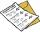The class has 22 students. 2 of which has assesement of 2. Count of assesement of 1 is three times more than count of students with assesement of 3. How many students has assesement of 1 and how many students has assesement of 1.
• There 12There are 42 students in the class and 2/3 of them are girls. How may girls are there in the class?
• StudentsIf the fifth-grade class left 20% of students and in the seventh grade were added two pupils, in the eighth one pupil, the number has not changed in the ninth grade, but it is now tenth students less than it was in the fifth grade. How many pupils are in
• Average height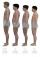In a class are 34 students. The average height of the students is 165 cm. What will be the average height of students in the classroom when two pupils, tall 176 cm and 170 cm, moved from this school/class? The average height in meters of all the students